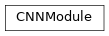# garage.torch.modules.cnn_module¶

CNN Module.

class CNNModule(input_var, hidden_channels, kernel_sizes, strides, hidden_nonlinearity=nn.ReLU, hidden_w_init=nn.init.xavier_uniform_, hidden_b_init=nn.init.zeros_, paddings=0, padding_mode='zeros', max_pool=False, pool_shape=None, pool_stride=1, layer_normalization=False, n_layers=None, is_image=True)

Bases: torch.nn.ModuleConvolutional neural network (CNN) model in pytorch.

Parameters
• input_var (pytorch.tensor) – Input tensor of the model. Based on ‘NCHW’ data format: [batch_size, channel, height, width].

• kernel_sizes (tuple[int]) – Dimension of the conv filters. For example, (3, 5) means there are two convolutional layers. The filter for first layer is of dimension (3 x 3) and the second one is of dimension (5 x 5).

• strides (tuple[int]) – The stride of the sliding window. For example, (1, 2) means there are two convolutional layers. The stride of the filter for first layer is 1 and that of the second layer is 2.

• hidden_channels (tuple[int]) – Number of output channels for CNN. For example, (3, 32) means there are two convolutional layers. The filter for the first conv layer outputs 3 channels and the second one outputs 32 channels.

• hidden_nonlinearity (callable or torch.nn.Module) – Activation function for intermediate dense layer(s). It should return a torch.Tensor. Set it to None to maintain a linear activation.

• hidden_w_init (callable) – Initializer function for the weight of intermediate dense layer(s). The function should return a torch.Tensor.

• hidden_b_init (callable) – Initializer function for the bias of intermediate dense layer(s). The function should return a torch.Tensor.

• paddings (tuple[int]) – Amount of zero-padding added to both sides of the input of a conv layer.

• padding_mode (str) – The type of padding algorithm to use, i.e. ‘constant’, ‘reflect’, ‘replicate’ or ‘circular’ and by default is ‘zeros’.

• max_pool (bool) – Bool for using max-pooling or not.

• pool_shape (tuple[int]) – Dimension of the pooling layer(s). For example, (2, 2) means that all pooling layers are of the same shape (2, 2).

• pool_stride (tuple[int]) – The strides of the pooling layer(s). For example, (2, 2) means that all the pooling layers have strides (2, 2).

• layer_normalization (bool) – Bool for using layer normalization or not.

• n_layers (int) – number of convolutional layer.

• is_image (bool) – Whether observations are images or not.

forward(self, input_val)

Forward method.

Parameters

input_val (torch.Tensor) – Input values with (N, C, H, W) shape.

Returns

Output values

Return type

List[torch.Tensor]